2012-11-07 21:13:15 jia20003 阅读数 9872
• ###### 学习OpenCV3.2+QT5+ffmpeg实战开发视频编辑器视频教程

OpenCV3.2+QT5+ffmpeg实战开发视频编辑器视频培训课程概况：教程中会讲解到基于opencv视频和摄像机录制、播放和播放进度控制，多视频图像合并、多视频图像融合、剪切、视频亮度、对比度、尺寸（近邻插值（手动实现），双线性插值，图像金字塔）、颜色格式（灰度图，二值化（阈值）），旋转镜像，视频裁剪（ROI），视频水印（ROI+weight），导出处理后的视频（包含音频，使用ffmpeg工具对音频进行抽取、剪切和终于opencv处理的视频合并）。

19572 人正在学习 去看看 夏曹俊

1.      三角函数基本知识，sin, cosin

2.      反三角函数基本知识，知道任意一点坐标P(x, y)求取该点的角度a = atag2(y/x)

3.      极坐标与笛卡尔坐标系转换知识1.      90度，180度，270度可以直接旋转坐标取得，像素直接映射取得。

2.      对于任何角度angle可以如下处理n = mod(angle, 90) = 1, 2, 3, 然后

将角度旋转90，180，270然后再旋转角度(angle– n * 90)。

1.      首先根据输入角度参数angle, 背景填充颜色bgcolor初始化

2.      计算出旋转以后的图像width与height

3.      循环每个输出像素，计算机坐标

4.      反旋转输入角度到输入的目标像素浮点数坐标

5.      使用双线性插值完成目标像素填充，如果不在范围之内填充背景色。

6.      得到输出像素数据，返回旋转后图像package com.gloomyfish.filter.study;

import java.awt.Color;
import java.awt.image.BufferedImage;
import java.awt.image.ColorModel;

public class RotateFilter extends AbstractBufferedImageOp {
private double angle;
private Color background;
private int outw;
private int outh;

public RotateFilter() {
this.angle = (45.0d/180.0d) * Math.PI;
background = Color.BLACK;
outw = -1;
outh = -1;
}

public void setDegree(double angle) {
this.angle = (angle/180.0d) * Math.PI;
}

public void setBackgroud(Color background) {
this.background = background;
}

public BufferedImage createCompatibleDestImage(BufferedImage src, ColorModel dstCM) {
if ( dstCM == null )
dstCM = src.getColorModel();
return new BufferedImage(dstCM, dstCM.createCompatibleWritableRaster(outw, outh), dstCM.isAlphaPremultiplied(), null);
}

@Override
public BufferedImage filter(BufferedImage src, BufferedImage dest) {
int width = src.getWidth();
int height = src.getHeight();

int[] inPixels = new int[width*height];
outw = (int)(width*Math.cos(angle)+height*Math.sin(angle));
outh = (int)(height*Math.cos(angle)+width*Math.sin(angle));
System.out.println("after rotate, new width : " + outw);
System.out.println("after rotate, new height: " + outh);

int[] outPixels = new int[outw*outh];
getRGB( src, 0, 0, width, height, inPixels );
int index = 0;

int centerPixel = inPixels[height/2 * width + width/2];

// calculate new center coordinate
float centerX = outw / 2.0f + 0.5f;
float centerY = outh /2.0f + 0.5f;

// calculate the original center coordinate
float ocenterX = width / 2.0f + 0.5f;
float ocenterY = height /2.0f + 0.5f;

float rx =0, ry = 0; //after rotated coordinate
float px = 0, py = 0; // original coordinate
float prow = 0, pcol = 0;
for(int row=0; row<outh; row++) {
for(int col=0; col<outw; col++) {
rx = col - centerX;
ry = centerY - row;
float fDistance = (float)Math.sqrt(rx * rx + ry * ry);
float fPolarAngle = 0; //;
if(rx != 0) {
fPolarAngle = (float)Math.atan2((double)ry, (double)rx);
} else {
if(rx == 0) {
if(ry == 0) {
outPixels[index] = centerPixel;
continue;
}
else if(ry < 0) {
fPolarAngle = 1.5f * (float)Math.PI;
} else {
fPolarAngle = 0.5f * (float)Math.PI;
}
}
}

// "reverse" rotate, so minus instead of plus
fPolarAngle -= angle;
px = fDistance * (float)Math.cos(fPolarAngle);
py = fDistance * (float)Math.sin(fPolarAngle);

// get original pixel float point
prow = ((float)ocenterY) - py;
pcol = ((float)ocenterX) + px;

// now start the biline-interpolation algorithm here!!!
int[] rgb = bilineInterpolation(inPixels, width, height, prow, pcol);

index = row * outw + col;
outPixels[index] = (255 << 24) | (rgb << 16) | (rgb << 8) | rgb;

}
}

if ( dest == null )
dest = createCompatibleDestImage( src, null );
setRGB( dest, 0, 0, outw, outh, outPixels );
return dest;
}

private int[] bilineInterpolation(int[] input, int width, int height, float prow, float pcol) {
double row = Math.floor(prow);
double col = Math.floor(pcol);
if(row < 0 || row >= height) {
return new int[]{background.getRed(), background.getGreen(), background.getBlue()};
}
if(col < 0 || col >= width) {
return new int[]{background.getRed(), background.getGreen(), background.getBlue()};
}

int rowNext = (int)row + 1, colNext = (int)col + 1;
if((row + 1) >= height) {
rowNext = (int)row;
}

if((col + 1) >= width) {
colNext = (int)col;
}
double t = prow - row;
double u = pcol - col;
double coffiecent1 = (1.0d-t)*(1.0d-u);
double coffiecent2 = (t)*(1.0d-u);
double coffiecent3 = t*u;
double coffiecent4 = (1.0d-t)*u;

int index1 = (int)(row * width + col);
int index2 = (int)(row * width + colNext);

int index3 = (int)(rowNext * width + col);
int index4 = (int)(rowNext * width + colNext);
int tr1, tr2, tr3, tr4;
int tg1, tg2, tg3, tg4;
int tb1, tb2, tb3, tb4;

tr1 = (input[index1] >> 16) & 0xff;
tg1 = (input[index1] >> 8) & 0xff;
tb1 = input[index1] & 0xff;

tr2 = (input[index2] >> 16) & 0xff;
tg2 = (input[index2] >> 8) & 0xff;
tb2 = input[index2] & 0xff;

tr3 = (input[index3] >> 16) & 0xff;
tg3 = (input[index3] >> 8) & 0xff;
tb3 = input[index3] & 0xff;

tr4 = (input[index4] >> 16) & 0xff;
tg4 = (input[index4] >> 8) & 0xff;
tb4 = input[index4] & 0xff;

int tr = (int)(tr1 * coffiecent1 + tr2 * coffiecent4 + tr3 * coffiecent2 + tr4 * coffiecent3);
int tg = (int)(tg1 * coffiecent1 + tg2 * coffiecent4 + tg3 * coffiecent2 + tg4 * coffiecent3);
int tb = (int)(tb1 * coffiecent1 + tb2 * coffiecent4 + tb3 * coffiecent2 + tb4 * coffiecent3);

return new int[]{tr, tg, tb};
}

}


2016-05-28 18:37:46 sinat_31261757 阅读数 5667
• ###### 学习OpenCV3.2+QT5+ffmpeg实战开发视频编辑器视频教程

OpenCV3.2+QT5+ffmpeg实战开发视频编辑器视频培训课程概况：教程中会讲解到基于opencv视频和摄像机录制、播放和播放进度控制，多视频图像合并、多视频图像融合、剪切、视频亮度、对比度、尺寸（近邻插值（手动实现），双线性插值，图像金字塔）、颜色格式（灰度图，二值化（阈值）），旋转镜像，视频裁剪（ROI），视频水印（ROI+weight），导出处理后的视频（包含音频，使用ffmpeg工具对音频进行抽取、剪切和终于opencv处理的视频合并）。

19572 人正在学习 去看看 夏曹俊2019-10-23 01:12:15 weixin_43981769 阅读数 27
• ###### 学习OpenCV3.2+QT5+ffmpeg实战开发视频编辑器视频教程

OpenCV3.2+QT5+ffmpeg实战开发视频编辑器视频培训课程概况：教程中会讲解到基于opencv视频和摄像机录制、播放和播放进度控制，多视频图像合并、多视频图像融合、剪切、视频亮度、对比度、尺寸（近邻插值（手动实现），双线性插值，图像金字塔）、颜色格式（灰度图，二值化（阈值）），旋转镜像，视频裁剪（ROI），视频水印（ROI+weight），导出处理后的视频（包含音频，使用ffmpeg工具对音频进行抽取、剪切和终于opencv处理的视频合并）。

19572 人正在学习 去看看 夏曹俊

# 数字图像处理实验——图像旋转

### 原理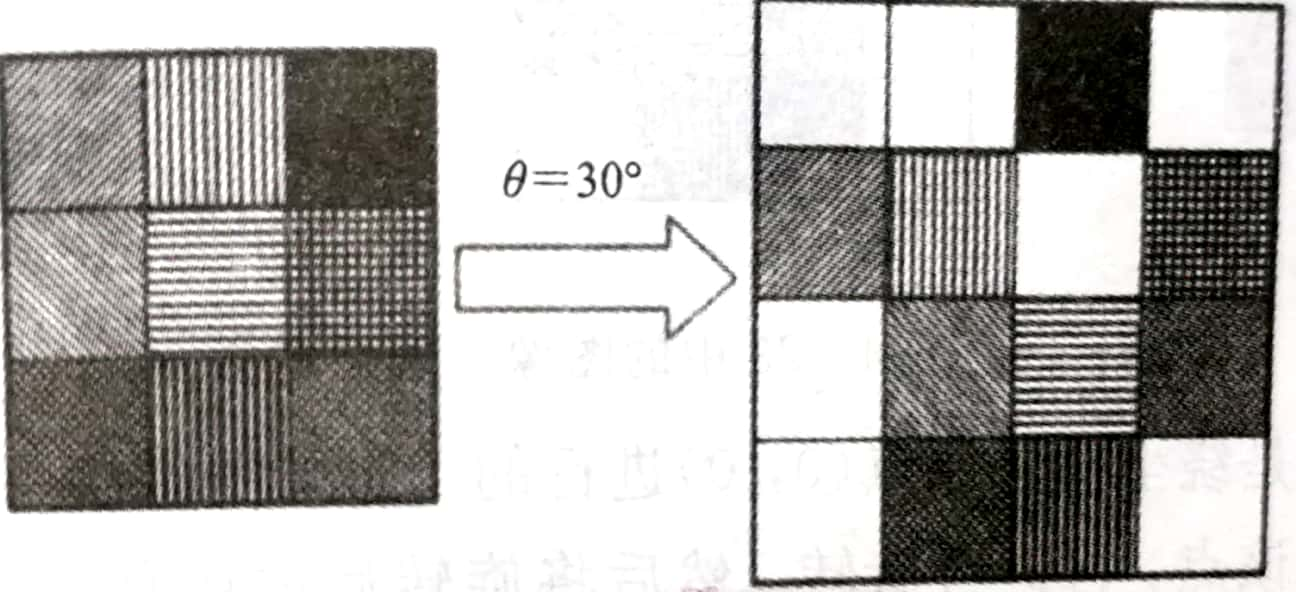### 前期准备

1. 旋转公式如下：(逆旋转将theta变为-theta即可)：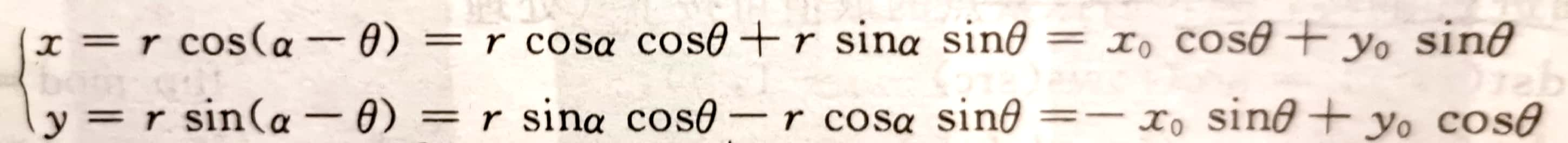2. 双线性插值：（其实就是与距离成反比）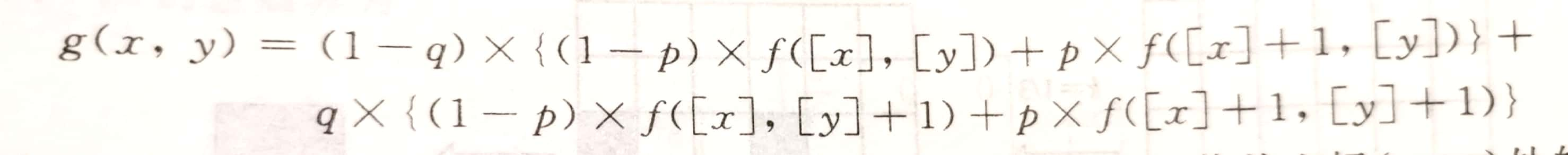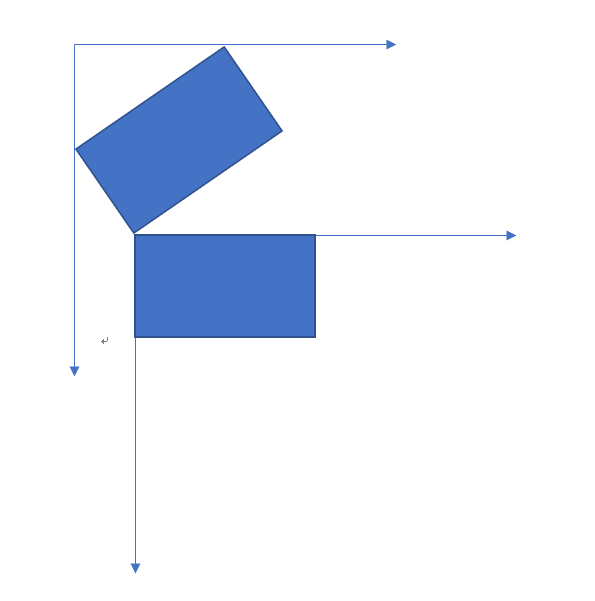X’ = X + dx，Y’ = Y + dy;

### 代码实现

#include <iostream>
#include <opencv2/opencv.hpp>
#include <cmath>

#define pi 3.1415926535
using namespace std;
using namespace cv;

/**
* @param img 待旋转图像
* @param angle 旋转角度，以逆时针旋转,单位为 度
*
* 此函数完成对图片旋转任意角度
*/
Mat imgRotate(Mat img, double angle);

/**
* 用于比较四个角点x,y坐标的最大最小值
* 返回值中前两个值为x方向的最大最小值
*/
Vec4d minmax(Point2d);

int main()
{
Mat rotation = imgRotate(test, 180);
imshow("original", test);
imshow("after rotation", rotation);
waitKey(0);
waitKey(0);
destroyAllWindows();
return 0;
}

Mat imgRotate(Mat img, double angle )
{
// 旋转公式为
// x = x0 * cos + y0 * sin
// y = -x0 * sin + y0 * cos

//逆变换为
// x0 = x * cos - y * sin
// y0 = y * cos + x * sin
// assert(!img.data);
angle *= pi / 180.0;  // 化为弧度
double rows = img.rows;
double cols = img.cols;
cout << rows << endl;
cout << cols << endl;
Point2d point = {Point2f(0, 0 ), Point2f(0 , cols - 1), Point2f(rows - 1, 0), Point2f(rows - 1, cols - 1)};  //定义四个角点,在新的坐标系下的坐标

for (int i = 0; i < 4; ++i)
{
double tempx = point[i].x;
double tempy = point[i].y;
point[i].x = tempx * cos(-angle) + tempy * sin(-angle);
point[i].y = -tempx * sin(-angle) + tempy * cos(-angle);
}
Vec4f min_max = minmax(point);
int width = abs((int)min_max) + abs((int)min_max) + 1 ;// 获得新坐标系的宽
int height = abs((int)min_max) + abs((int)min_max) + 1; // 获得新坐标系的高

cout << width << endl;
cout << height << endl;

int dx = min_max;
int dy = min_max;

Mat afterrotate(height, width,CV_8U, Scalar(0));

for (int i = 0; i < afterrotate.rows; ++i)
{
for (int j = 0; j < afterrotate.cols; ++j)
{
double tempx = ((i + dx)* cos(angle) + (j + dy) * sin(angle)) ; // 新图像逆旋转后在旧坐标系下的坐标
double tempy = (-(i + dx) * sin(angle) + (j + dy) * cos(angle));

if((int)tempx < 0 || (int)tempx >= img.rows)
{

}
else if((int)tempy < 0 || (int)tempy >= img.cols)
{

}
else
{
double x_distance = tempx - int(tempx);// 记录小数部分,也即距离
double y_distance = tempy - int(tempy);
afterrotate.at<uchar>(i , j) = (1 - y_distance) *((1 - x_distance) * (int)img.at<uchar>((int)tempx, (int)tempy) + x_distance * (int)img.at<uchar>((int)tempx + 1, (int)tempy)) + y_distance * (
(1 - x_distance) * (int)img.at<uchar>((int)tempx, (int)tempy + 1) + x_distance * (int)img.at<uchar>((int)tempx + 1, (int)tempy + 1)
);
}
}
}

return afterrotate;
}

Vec4d minmax(Point2d a)
{
Vec4f temp;
float x_max = a.x;
float x_min = a.x;

float y_max = a.y;
float y_min = a.y;
for(int i = 0; i < 4; ++i)
{
if(a[i].x > x_max)
x_max = a[i].x;

if(a[i].x < x_min)
x_min = a[i].x;

if(a[i].y > y_max)
y_max = a[i].y;

if(a[i].y < y_min)
y_min = a[i].y;
}
temp = x_max;
temp = x_min;
temp = y_max;
temp = y_min;

cout << temp << endl;
return temp;
}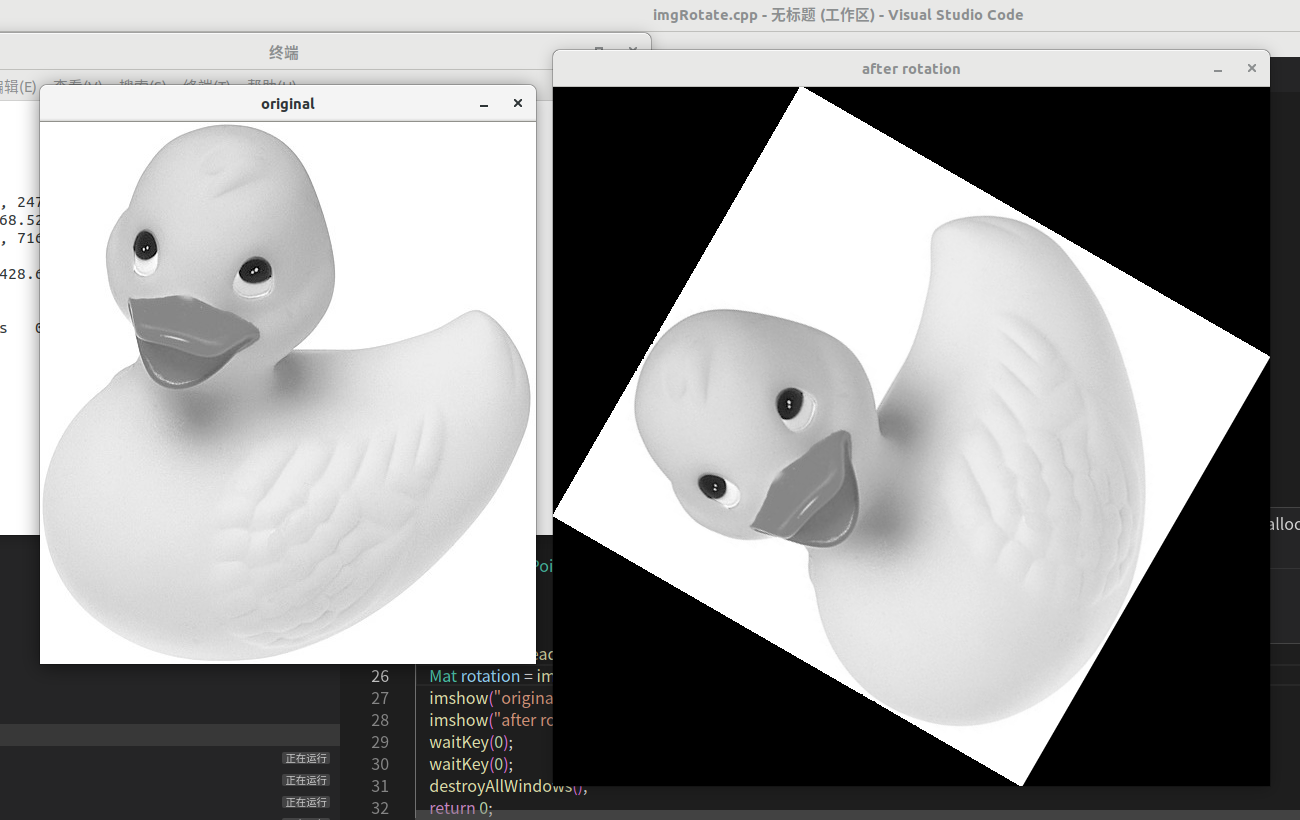### 总结

2019-01-27 21:35:08 qq_39475211 阅读数 272
• ###### 学习OpenCV3.2+QT5+ffmpeg实战开发视频编辑器视频教程

OpenCV3.2+QT5+ffmpeg实战开发视频编辑器视频培训课程概况：教程中会讲解到基于opencv视频和摄像机录制、播放和播放进度控制，多视频图像合并、多视频图像融合、剪切、视频亮度、对比度、尺寸（近邻插值（手动实现），双线性插值，图像金字塔）、颜色格式（灰度图，二值化（阈值）），旋转镜像，视频裁剪（ROI），视频水印（ROI+weight），导出处理后的视频（包含音频，使用ffmpeg工具对音频进行抽取、剪切和终于opencv处理的视频合并）。

19572 人正在学习 去看看 夏曹俊

# 常用的插值方法

## 双线性插值$f( i+u, j+v)=(1-u)(1-v) f(i,j) + (1-u) v f(i,j+1) + u (1-v) f( i+1,j ) + u v f( i+1, j+1)$

# 常见的映射方法

## 前向映射（前向插值）1. 浮点坐标：如（1,1）映射成（0.5,0.5），这是无效坐标，这是可以用插值计算法进一步处理；
2. 映射不完全：输入图像的像素总数小于输出的像素总数，会使得输出图像的部分像素与原始图像并没有映射关系，如放大操作；
3. 映射重叠：与映射不完全正好相反，输出图像可能会存在映射后的像素重叠。

## 后向映射# 图像旋转方法

$\begin{bmatrix} {x_{1}}&{y_{1}}&{1}\\ \end{bmatrix}$ = $\begin{bmatrix} {x_{0}}&{y_{0}}&{1}\\ \end{bmatrix}$ $\begin{bmatrix} {cos(\theta)}&{sin(\theta)}&{0}\\ {-sin(\theta)}&{cos(\theta)}&{0}\\ {0)}&{0}&{1}\\ \end{bmatrix}$

$\begin{bmatrix} {x_{0}}&{y_{0}}&{1}\\ \end{bmatrix}$ = $\begin{bmatrix} {x_{1}}&{y_{1}}&{1}\\ \end{bmatrix}$ $\begin{bmatrix} {cos(\theta)}&{-sin(\theta)}&{0}\\ {sin(\theta)}&{cos(\theta)}&{0}\\ {0)}&{0}&{1}\\ \end{bmatrix}$

# 小实验示例代码

1. 读取cameraman.tif，自行编写代码，分别使用前向插值（使用最邻近法），后向插值（自己选择合适的插值方法），将图像绕左上角逆时针旋转30度，再顺时针旋转30度，显示并计算旋转前后图像的MSE，分析原因。clc
clear;
[r1,c1]=size(I);
J = uint8(myimrotatef(I,30));%rotate 30 degrees clockwise
K = uint8(myimrotatef(J,-30));%rotate 30 degrees anticlockwise
[r2,c2]=size(K);
K = K((r2-r1)/2+1:(r2+r1)/2,(c2-c1)/2+1:(c2+c1)/2);%cut the picture as large as the sample
subplot(1,3,1),imshow(I),title("Sample 256*256"),
subplot(1,3,2),imshow(J),title("Rotation1 350*350"),
subplot(1,3,3),imshow(K),title("Rotation2 256*256");
[PSNR, MSE] = psnr_mse(I, K);%calculate MSE and display MSE


by Morol_
cambridge.mo@foxmail.com

2017-10-20 18:41:19 u010385790 阅读数 1403
• ###### 学习OpenCV3.2+QT5+ffmpeg实战开发视频编辑器视频教程

OpenCV3.2+QT5+ffmpeg实战开发视频编辑器视频培训课程概况：教程中会讲解到基于opencv视频和摄像机录制、播放和播放进度控制，多视频图像合并、多视频图像融合、剪切、视频亮度、对比度、尺寸（近邻插值（手动实现），双线性插值，图像金字塔）、颜色格式（灰度图，二值化（阈值）），旋转镜像，视频裁剪（ROI），视频水印（ROI+weight），导出处理后的视频（包含音频，使用ffmpeg工具对音频进行抽取、剪切和终于opencv处理的视频合并）。

19572 人正在学习 去看看 夏曹俊

# 关于图像处理中的插值和旋转

【将点一次带入得到方程组/最小二乘法列出方程组】voidfittingCurve3D(double *x,double *y,double *z,intn,double *index)

{

//直接把点带入方程，把方程组写成矩阵形式，结果用伪逆表示[inv（A'A）]A'b

double *a =newdouble[n * 6];//已知点的信息矩阵,含xy

double *b =newdouble[n];//已知点的信息矩阵,含z

///////构造a的一维数组,n*6

for (int i = 0; i <n * 6; ++i)

{

if (i % 6 == 0)

{

a[i]= 1;

a[i+ 1] = x[i / 6];//x

a[i+ 2] = y[i / 6];//y

a[i+ 3] = x[i / 6] * x[i / 6];//x^2

a[i+ 4] = y[i / 6] * y[i / 6];//y^2

a[i+ 5] = x[i / 6] * y[i / 6];//x*y

}

}

CvMat AMat = cvMat(n, 6,CV_64FC1, a);//变成矩阵AMat

CvMat *ATransposedMat =cvCreateMat(AMat.cols, AMat.rows,CV_64FC1);

cvTranspose(&AMat,ATransposedMat);//转置ATransposedMat

///用于存储矩阵相乘结果，构造的数组n*n

double *c =newdouble[6 * 6];

for (int i = 0; i < 6 *6; ++i)

{

c[i]= 0;

}

CvMat invMat1 = cvMat(6,6,CV_64FC1, c);//变成矩阵invMat1

cvGEMM(ATransposedMat,&AMat, 1, NULL, 0,&invMat1, 0);// ATransposedMat(转置)*AMat=invMat1

cvInvert(&invMat1,&invMat1, 0);//求invMat1的逆矩阵,invMat1

///////构造b的一维数组,n*1

for (int i = 0; i <n; ++i)

{

b[i]= z[i];

}

CvMat BMat = cvMat(n, 1,CV_64FC1, b);//变成矩阵BMat

//用于存储矩阵相乘结果，构造的数组6*1

double *d =newdouble;

for (int i = 0; i < 6;++i)

{

d[i]= 0;

}

CvMat invMat2 = cvMat(6,1,CV_64FC1, d);//变成矩阵invMat2

cvGEMM(ATransposedMat,&BMat, 1, NULL, 0,&invMat2, 0);//AMat的转置矩阵 * BMat矩阵 = invMat2

CvMat indexMat = cvMat(6,1,CV_64FC1, index);

cvGEMM(&invMat1,&invMat2, 1, NULL, 0,&indexMat, 0);//invMat1 * invMat2 = indexMat

cvReleaseMat(&ATransposedMat);

delete[] a;

delete[] b;

delete[] c;

delete[] d;

}

http://blog.csdn.net/hj199404182515/article/details/59480954

double xtemp0 = c*x - s*y + (-0.5*c*(srcW - 1) + 0.5*s*(srcH - 1) +0.5*dstW);

double ytemp0 = s*x + c*y + (-0.5*s*(srcW - 1) -0.5*c*(srcH - 1) + 0.5*dstH);

double xtemp = c*i + s*j + (-c*(dstW - 1)*0.5 - s* (dstH - 1)*0.5+0.5*temp->width);

double ytemp = -s*i + c*j + (s*(dstW - 1)*0.5 - c* (dstH -1)*0.5+ 0.5*temp->height);

//将原图复制到临时图像temp中心  (保证原图可以任意角度旋转的最小尺寸 )

int tempLength =int( sqrt((double)width *width + (double)height *height) + 10);

int tempX = (tempLength + 1) / 2 - width /2;

int tempY = (tempLength + 1) / 2 - height /2;

IplImage* temp =cvCreateImage(cvSize(tempLength, tempLength), src->depth,src->nChannels);

memset(temp->imageData, 255,  sizeof(char)*temp->imageSize);

cvSetImageROI(temp, cvRect(tempX, tempY,width, height));

cvCopy(src, temp, NULL);

cvResetImageROI(temp);

cvReleaseImage(&temp);

http://www.cnblogs.com/mlv5/archive/2012/02/02/2336321.html

intImgBilinearInter(floatfx,floatfy,IplImage * img) {

//参考函数cv::PicRotaryBilInear——双线性插值

int value;

int x = floor(fx);

int y = floor(fy);

cv::Point P0 = Pixels_Bound(img, x, y);

cv::Point P2 = Pixels_Bound(img, x + 1, y);

cv::Point P1 = Pixels_Bound(img, x, y + 1);

cv::Point P3 = Pixels_Bound(img, x + 1, y + 1);//边界饱和

float u =fx - x;

float v =fy - y;

float pm3 = u*v;

float pm2 = u*(1 - v);

float pm1 = v*(1 - u);

float pm0 = (1 - u)*(1 -v);

// 指向图像的指针

uchar* lp = (uchar *)img->imageData;

int step =img->widthStep /sizeof(uchar);

//value = (pm0*lp[P0.y*step + P0.x] + pm1*lp[P1.y *step +P1.x] + pm2*lp[P2.y *step + P2.x ] + pm3*lp[P3.y *step + P3.x ]);

float value_float =(pm0*lp[P0.y*step + P0.x] + pm1*lp[P1.y *step + P1.x] + pm2*lp[P2.y *step +P2.x] + pm3*lp[P3.y *step + P3.x]);

value= round(value_float);

return value;

}

cv::Point Pixels_Bound(IplImage *pic, intx0,inty0) {

if (x0<0) {x0 = 0; }

elseif (x0 >=pic->width) { x0 = pic->width - 1; }

if (y0<0) {y0 = 0; }

elseif (y0 >=pic->height) { y0 = pic->height - 1; }

cv::Point P(x0,y0);

return P;

}